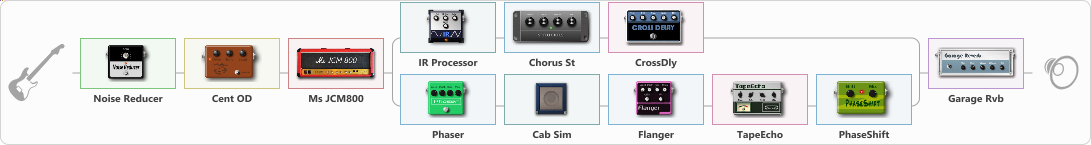# Eddy Vh truss

Discussion in 'ToneLib-GFX presets' started by truss van halen, Oct 2, 2020.

1. ### truss van halenWell-Known Member

Eddy Vh truss

Preset name: EDDY VH truss

Effects chain:Effect: "Noise Reducer" (Dynamics / Filter), active - "yes"
{
"Sens" = 100
"Mode" = Hard
}

Effect: "Cent OD" (Overdrive / Distortion), active - "yes"
{
"Drive" = 52
"Tone" = 100
"Level" = 74
}

Effect: "Ms JCM800" (Amp simulators), active - "yes"
{
"Gain" = 73
"Bass" = 21
"Middle" = 58
"Treble" = 60
"Presence" = 65
"Master" = 49
"Level (dB)" = 0
}

Effect: "Splitter" (Dynamics / Filter), active - "yes"
{
"A-Bypass" = Off
"A-Pan" = -42
"A-Level" = 55
"B-Bypass" = Off
"B-Pan" = 50
"B-Level" = 55

'A' branch:
{

Effect: "IR Processor" (Cabinets), active - "yes"
{
"IR" = Cab IR Sample3
"Low Cut (Hz)" = 0
"Hi Cut (kHz)" = 11.5
"Mix" = 100
"Level (dB)" = 10
}

Effect: "Chorus St" (Modulation / Sfx), active - "yes"
{
"Speed" = 1.9
"Depth" = 31
"Center" = 4.4
"Mix" = 40
}

Effect: "CrossDly" (Delay), active - "yes"
{
"Time" = 756
"Feedback" = 27
"Tone" = 57
"Sens" = 29
"Mix" = 34
}
}
'B' branch:
{

Effect: "Phaser" (Modulation / Sfx), active - "yes"
{
"Model" = MXR Phase 90
"Speed" = 2.0
"Depth" = 66
"Reso" = 19
"Freq" = 5.0
}

Effect: "Cab Sim" (Cabinets), active - "yes"
{
"Model" = 4x12" 1960 T75
"Level (dB)" = 7
}

Effect: "Flanger" (Modulation / Sfx), active - "yes"
{
"Speed" = 2.2
"Depth" = 71
"Reso" = 35
"Center" = 7.7
"Offset" = 0.0
"Mix" = 57
"Reset" = On
}

Effect: "TapeEcho" (Delay), active - "yes"
{
"Time" = 257
"Feedback" = 38
"Tone" = 65
"LoDamp" = 19
"Mix" = 65
}

Effect: "PhaseShift" (Modulation / Sfx), active - "yes"
{
"Shift" = 50
"Mix" = 64
}
}
}

Effect: "Garage Rvb" (Reverberation), active - "yes"
{
"Time" = 3.3
"PreDelay" = 29
"LoDamp" = 0
"HiDamp" = 30
"Mix" = 61
}

Note: You will need to download and install the ToneLib-GFX software to use the preset.

#### Attached Files:

• ###### Eddy_Vh_truss.tlgfx
File size:
2.9 KB
Views:
2,654
SEMENOV, Jack Disney, stroebs and 5 others like this.